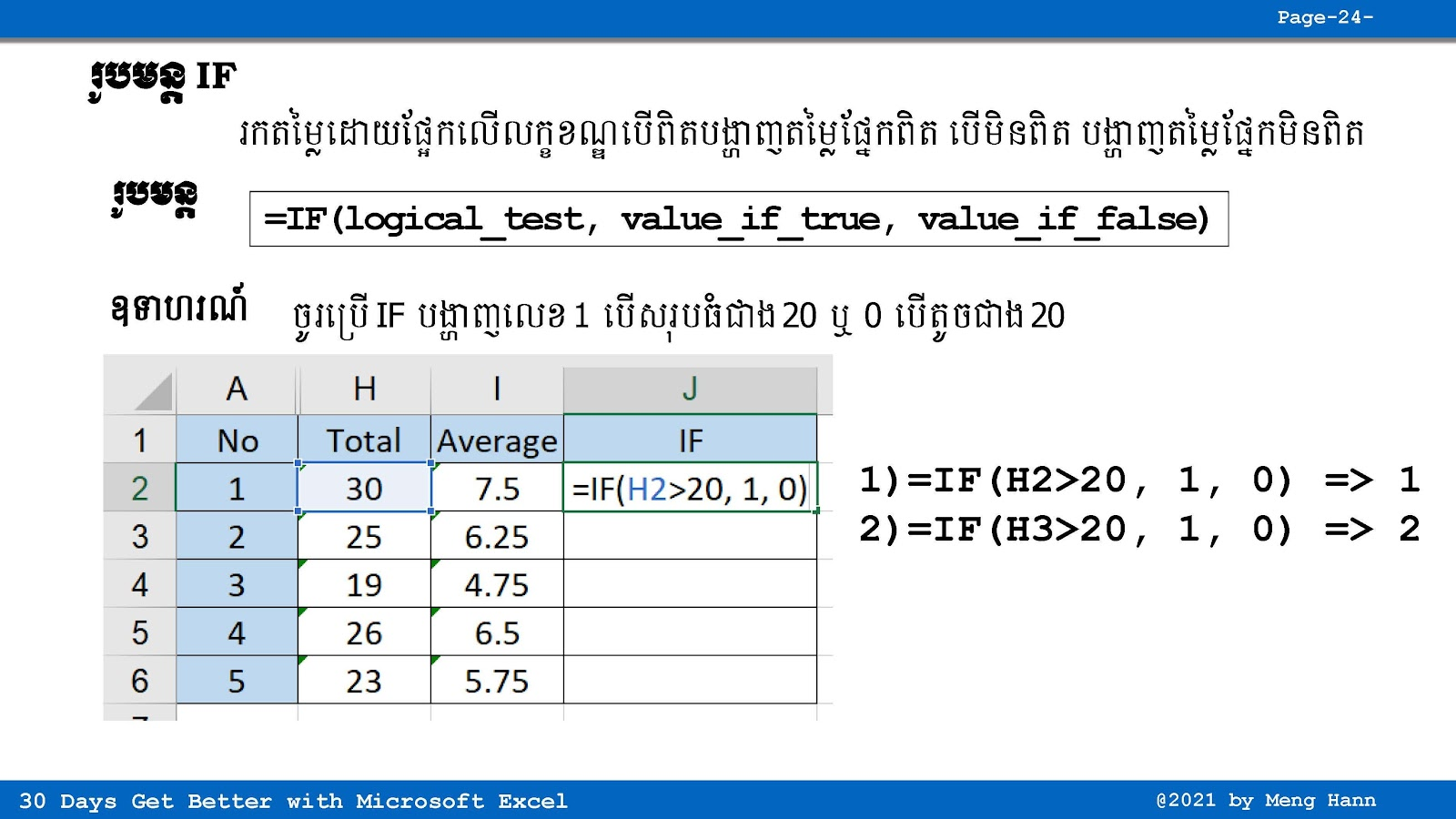# 25. How to use IF formula in Excel speak Khmer

25. How to use IF formula in Excel speak Khmer
If formula is working base on condition, If the condition is True, then do something (True Part), if not do something else instead (Fail Part). IF Formula:
=IF(Something is True, then do something, otherwise do something else)How to use Comparision Condition in Excel